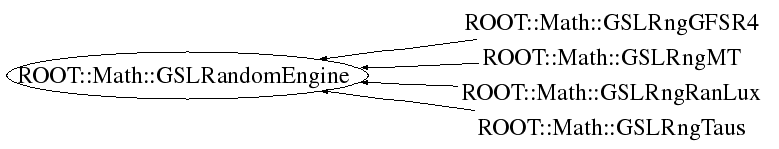# class ROOT::Math::GSLRandomEngine

```
GSLRandomEngine
Base class for all GSL random engines,
normally user instantiate the derived classes
which creates internally the generator.

The main GSL generators (see
<A HREF="http://www.gnu.org/software/gsl/manual/html_node/Random-number-generator-algorithms.html">
here</A>) are available as derived classes
In addition to generate uniform numbers it provides method for
generating numbers according to pre-defined distributions
using the GSL functions from
<A HREF="http://www.gnu.org/software/gsl/manual/html_node/Random-Number-Distributions.html">
GSL random number distributions</A>.

@ingroup Random

```

## Function Members (Methods)

public:
 virtual ~GSLRandomEngine() unsigned int Binomial(double p, unsigned int n) const double Cauchy(double a) const double ChiSquare(double nu) const void Dir2D(double& x, double& y) const void Dir3D(double& x, double& y, double& z) const double Exponential(double mu) const double FDist(double nu1, double nu2) const double Gamma(double a, double b) const double Gaussian(double sigma) const void Gaussian2D(double sigmaX, double sigmaY, double rho, double& x, double& y) const double GaussianRatio(double sigma) const double GaussianTail(double a, double sigma) const double GaussianZig(double sigma) const ROOT::Math::GSLRandomEngine GSLRandomEngine() ROOT::Math::GSLRandomEngine GSLRandomEngine(ROOT::Math::GSLRngWrapper* rng) ROOT::Math::GSLRandomEngine GSLRandomEngine(const ROOT::Math::GSLRandomEngine&) void Initialize() double Landau() const double LogNormal(double zeta, double sigma) const vector Multinomial(unsigned int ntot, const vector& p) const string Name() const double operator()() const ROOT::Math::GSLRandomEngine& operator=(const ROOT::Math::GSLRandomEngine&) unsigned int Poisson(double mu) const void RandomArray(double* begin, double* end) const unsigned int RndmInt(unsigned int max) const void SetSeed(unsigned int seed) const unsigned int Size() const double tDist(double nu) const void Terminate()

## Data Members

private:
 unsigned int fCurTime current time used to seed the generator ROOT::Math::GSLRngWrapper* fRng pointer to GSL generator wrapper

## Class Charts## Function documentation

```default constructor. No creation of rng is done.
If then Initialize() is called an engine is created
based on default GSL type (MT)

```
GSLRandomEngine( GSLRngWrapper * rng)
```create from an existing rng.
User manage the rng pointer which is then deleted olny by calling Terminate()

```
`{}`
void Initialize()
```initialize the generator
If no rng is present the default one based on Mersenne and Twister is created

```
void Terminate()
```delete pointer to contained rng

```
virtual ~GSLRandomEngine()
```no operation, rng is delete in Terminate()

```
`{}`
double operator()()
```Generate a  random number between ]0,1]
0 is excluded and 1 is included

```
unsigned int RndmInt(unsigned int max) const
```Generate an integer number between [0,max-1] (including 0 and max-1)
if max is larger than available range of algorithm
an error message is printed and zero is returned

```
void RandomArray(double* begin, double* end) const
```Generate an array of random numbers.
The iterators points to the random numbers

```
std::string Name()
```eturn name of generato

```
unsigned int Size()
```eturn the state size of generato

```
void SetSeed(unsigned int seed) const
```set the random generator seed

```
double Gaussian(double sigma) const
``` @name Random Distributions
Implemented using the
<A HREF="http://www.gnu.org/software/gsl/manual/html_node/Random-Number-Distributions.html">
GSL Random number Distributions</A>

@{

Gaussian distribution - default method is Box-Muller (polar method)

```
double GaussianZig(double sigma) const
```Gaussian distribution - Ziggurat method

```
double GaussianRatio(double sigma) const
```Gaussian distribution - Ratio method

```
double GaussianTail(double a, double sigma) const
void Gaussian2D(double sigmaX, double sigmaY, double rho, double& x, double& y) const
```Bivariate Gaussian distribution with correlation

```
double Exponential(double mu) const
```Exponential distribution

```
double Cauchy(double a) const
```Cauchy distribution

```
double Landau()
```Landau distribution

```
double Gamma(double a, double b) const
```Gamma distribution

```
double LogNormal(double zeta, double sigma) const
```Log Normal distribution

```
double ChiSquare(double nu) const
```Chi square distribution

```
double FDist(double nu1, double nu2) const
```F distrbution

```
double tDist(double nu) const
```t student distribution

```
void Dir2D(double& x, double& y) const
```generate random numbers in a 2D circle of radious 1

```
void Dir3D(double& x, double& y, double& z) const
```generate random numbers in a 3D sphere of radious 1

```
unsigned int Poisson(double mu) const
```Poisson distribution

```
unsigned int Binomial(double p, unsigned int n) const
```Binomial distribution

```
std::vector<unsigned int> Multinomial(unsigned int ntot, const vector<double>& p) const
```Multinomial distribution

```

Author: L. Moneta, A. Zsenei 08/2005
Last update: root/mathmore:\$Id: GSLRndmEngines.h 21553 2007-12-21 10:55:46Z moneta \$
Copyright (c) 2004 ROOT Foundation, CERN/PH-SFT *

This page has been automatically generated. If you have any comments or suggestions about the page layout send a mail to ROOT support, or contact the developers with any questions or problems regarding ROOT.